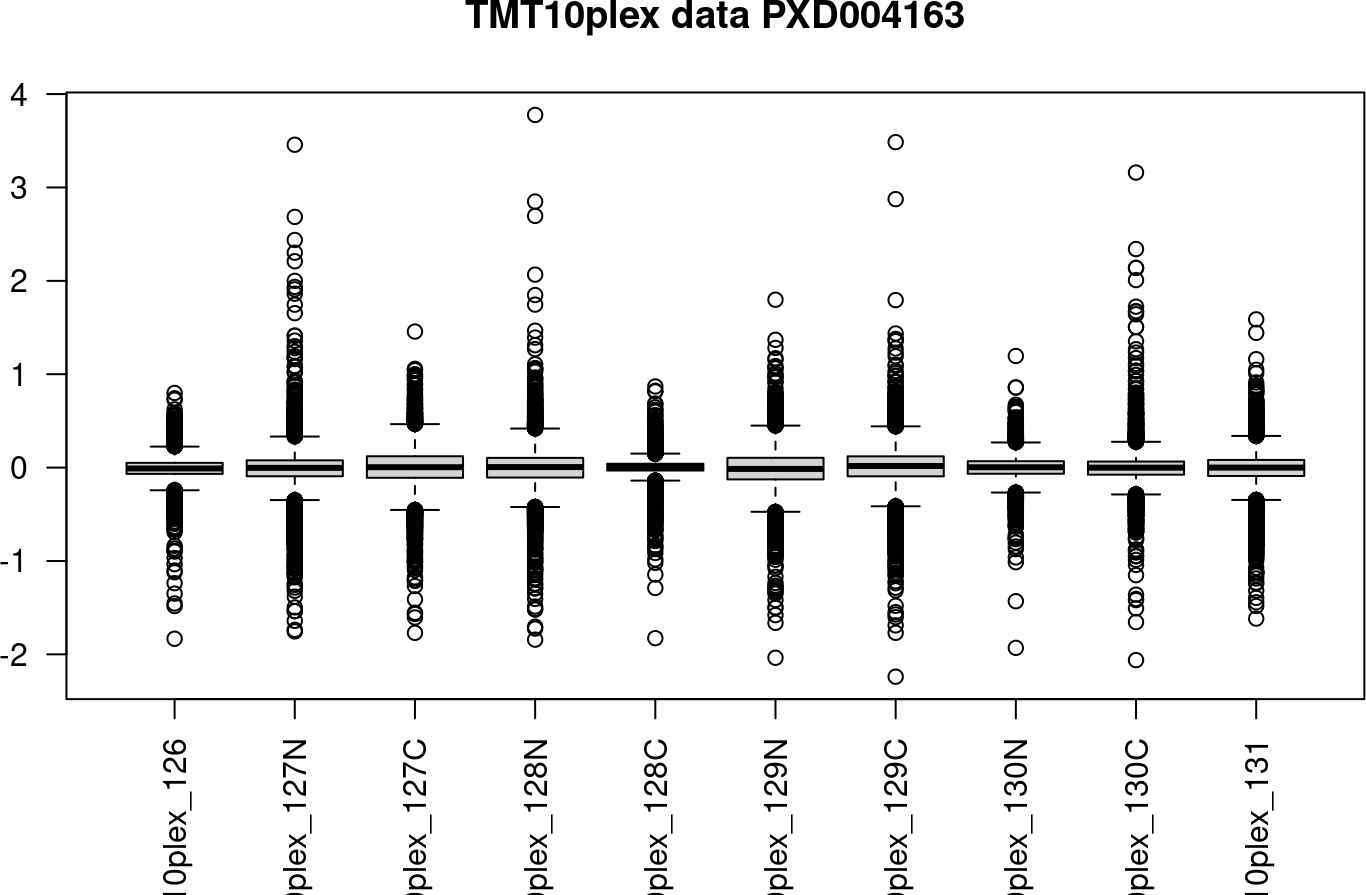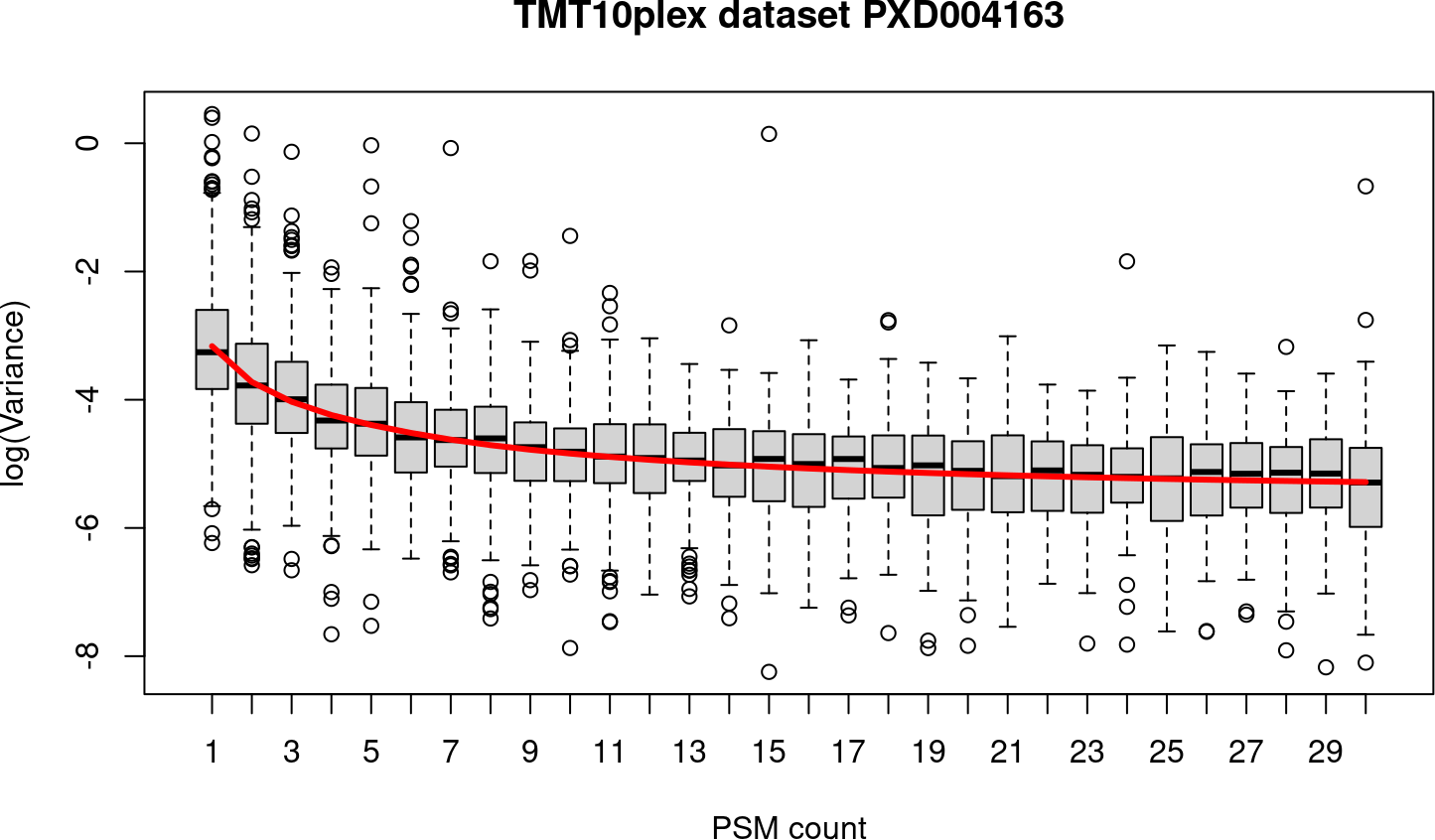# 1 Overview of DEqMS

`DEqMS` builds on top of `Limma`, a widely-used R package for microarray data analysis (Smyth G. et al 2004), and improves it with proteomics data specific properties, accounting for variance dependence on the number of quantified peptides or PSMs for statistical testing of differential protein expression.

Limma assumes a common prior variance for all proteinss, the function `spectraCounteBayes` in DEqMS package estimate prior variance for proteins quantified by different number of PSMs.

A documentation of all R functions available in DEqMS is detailed in the PDF reference manual on the DEqMS Bioconductor page.

``library(DEqMS)``
``## Loading required package: ggplot2``
``## Loading required package: matrixStats``
``## Loading required package: limma``

# 2 Quick start

## 2.1 Differential protein expression analysis with DEqMS using a protein table

As an example, we analyzed a protemoics dataset (TMT10plex labelled) in which A431 cells (human epidermoid carcinoma cell line) were treated with three different miRNA mimics (Zhou Y. Et al Oncogene 2017). The raw MS data was searched with MS-GF+ (Kim et al Nat Communications 2016) and post processed with Percolator (Kall L. et al Nat Method 2007). A tabular text output of protein table filtered at 1% protein level FDR is used.

``````url <- "https://ftp.ebi.ac.uk/pride-archive/2016/06/PXD004163/Yan_miR_Protein_table.flatprottable.txt"

header = TRUE, quote = "", comment.char = "",sep = "\t")``````

### 2.1.2 Extract quant data columns for DEqMS

``````# filter at 1% protein FDR and extract TMT quantifications
TMT_columns = seq(15,33,2)
dat = df.prot[df.prot\$miR.FASP_q.value<0.01,TMT_columns]
rownames(dat) = df.prot[df.prot\$miR.FASP_q.value<0.01,]\$Protein.accession
# The protein dataframe is a typical protein expression matrix structure
# Samples are in columns, proteins are in rows
# use unique protein IDs for rownames
# to view the whole data frame, use the command View(dat)``````

If the protein table is relative abundance (ratios) or intensity values, Log2 transform the data. Systematic effects and variance components are usually assumed to be additive on log scale (Oberg AL. et al JPR 2008; Hill EG. et al JPR 2008).

``````dat.log = log2(dat)
#remove rows with NAs
dat.log = na.omit(dat.log)``````

Use boxplot to check if the samples have medians centered. if not, do median centering.

``boxplot(dat.log,las=2,main="TMT10plex data PXD004163")````````# Here the data is already median centered, we skip the following step.
# dat.log = equalMedianNormalization(dat.log)``````

### 2.1.3 Make design table.

A design table is used to tell how samples are arranged in different groups/classes.

``````# if there is only one factor, such as treatment. You can define a vector with
# the treatment group in the same order as samples in the protein table.
cond = as.factor(c("ctrl","miR191","miR372","miR519","ctrl",
"miR372","miR519","ctrl","miR191","miR372"))

# The function model.matrix is used to generate the design matrix
design = model.matrix(~0+cond) # 0 means no intercept for the linear model
colnames(design) = gsub("cond","",colnames(design))``````

### 2.1.4 Make contrasts

In addition to the design, you need to define the contrast, which tells the model to compare the differences between specific groups. Start with the Limma part.

``````# you can define one or multiple contrasts here
x <- c("miR372-ctrl","miR519-ctrl","miR191-ctrl",
"miR372-miR519","miR372-miR191","miR519-miR191")
contrast =  makeContrasts(contrasts=x,levels=design)
fit1 <- lmFit(dat.log, design)
fit2 <- contrasts.fit(fit1,contrasts = contrast)
fit3 <- eBayes(fit2)``````

### 2.1.5 DEqMS analysis

The above shows Limma part, now we use the function `spectraCounteBayes` in DEqMS to correct bias of variance estimate based on minimum number of psms per protein used for quantification.We use the minimum number of PSMs used for quantification within and across experiments to model the relation between variance and PSM count.(See original paper)

``````# assign a extra variable `count` to fit3 object, telling how many PSMs are
# quantifed for each protein
library(matrixStats)
count_columns = seq(16,34,2)
psm.count.table = data.frame(count = rowMins(
as.matrix(df.prot[,count_columns])), row.names =  df.prot\$Protein.accession)
fit3\$count = psm.count.table[rownames(fit3\$coefficients),"count"]
fit4 = spectraCounteBayes(fit3)``````

Outputs of `spectraCounteBayes`:
object is augmented form of “fit” object from `eBayes` in Limma, with the additions being:
`sca.t` - Spectra Count Adjusted posterior t-value
`sca.p` - Spectra Count Adjusted posterior p-value
`sca.dfprior` - DEqMS estimated prior degrees of freedom
`sca.priorvar`- DEqMS estimated prior variance
`sca.postvar` - DEqMS estimated posterior variance
`model` - fitted model

### 2.1.6 Visualize the fit curve - variance dependence on quantified PSM

``````# n=30 limits the boxplot to show only proteins quantified by <= 30 PSMs.
VarianceBoxplot(fit4,n=30,main="TMT10plex dataset PXD004163",xlab="PSM count")````````VarianceScatterplot(fit4,main="TMT10plex dataset PXD004163")``